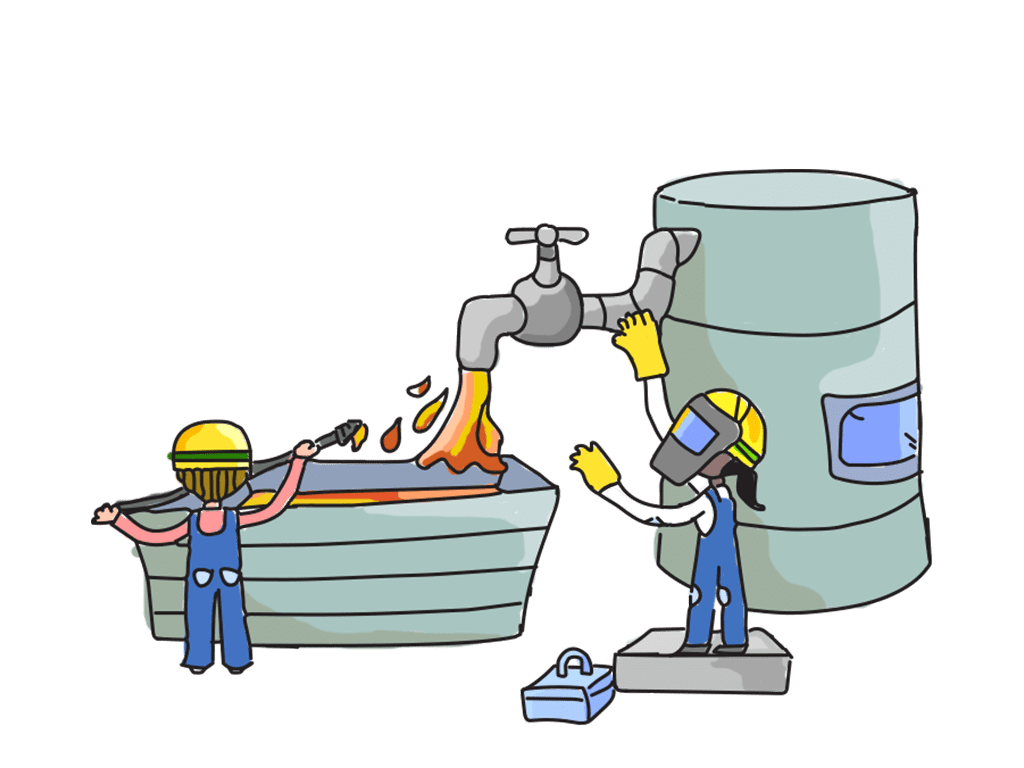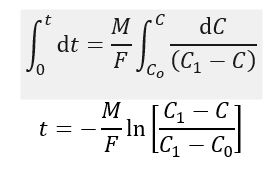# Material Balances involving Unsteady-State Process

In previous posts, I shared how to calculate material balances of several cases. Those calculation involve steady-state condition. In steady-state process, the accumulation is zero. The streams flowrate and composition are also did not vary with time. The calculation are more complex if these conditions are not met. The unsteady-state behaviour of a process is important when considering the process start-up and shut-down, and the response to process upsets.

Unsteady-state material balances are solved by setting up balances over a small increment of time, which results in a set of differential equations that describe the process. These equations can be solved analytically for simple problems. Computer-based methods would be employed for more difficult problems.

Example below is an example of general approach used to solve un-steady state problem.

Example

A hold tank is installed in an aqueous effluent-treatment process to smooth out fluctuations in concentration in the effluent stream. The effluent feed to the tank normally contains no more than 80 ppm of acetone. The maximum allowable concentration of acetone in the effluent discharge is set at 200 ppm. The surge tank working capacity is 600 m3 and it can be considered to be perfectly mixed. The effluent flow is 50,000 kg/h. If the acetone concentration in the feed suddenly rises to 1200 ppm, due to a spill in the process plant, and stays at that level for half an hour, will the limit of 200 ppm in the effluent discharge be exceeded?

Solution

Basis: increment of time ∆t

The balance will be set up in terms of symbols for all the quantities and then actual values for this example substituted.

Let,

Material in the tank = M

Initial concentration in the tank = C0

Concentration at time t after the feed concentration is increased = C

Concentration in the effluent feed = 1

­Change in concentration over time increment ∆t = ∆C

Average concentration in the tank during the time increment = Cav

In this post, the conservation of mass equation is:

Material in + Generated – Consumption – Accumulation = Material Out

Since, there is no reaction, then material generated and consumed are zero. Then, the general material balance equation becomes:

Material in – Material Out = Accumulation

As the tank is considered to be perfectly mixed, the outlet concentration will be the same as the concentration in the tank.

Material balance on acetone:

Acetone in – Acetone out = Acetone accumulated in the tank

F C1t  – F Cavt = M (C + ∆C) – M C

F (C1Cav) = M

Taking the limit ∆t → 0,Integrating,Substituting the values for the example, noting that the maximum outlet concentration will occur at the end of the half-hour period of high inlet concentration.

t = 0.5 h

C1 = 1200 ppm

C0 = 80 ppm (normal value)

M = 600 m3 = 600,000 kg

F = 50,000 kg/hSo, the maximum allowable concentration will not be exceed.